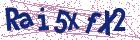# Modified Runge–Kutta method with convergence analysis for nonlinear stochastic differential equations with Hölder continuous diffusion coeﬀicient

Document Type : Research Article

Author

Department of Mathematics, Faculty of Science, Razi University, Kermanshah 67149, Iran.

Abstract

The main goal of this work is to develop and analyze an accurate trun-cated stochastic Runge–Kutta (TSRK2) method to obtain strong numeri-cal solutions of nonlinear one-dimensional stochastic differential equations (SDEs) with continuous Hölder diffusion coeﬀicients. We will establish the strong L1-convergence theory to the TSRK2 method under the local Lipschitz condition plus the one-sided Lipschitz condition for the drift co-eﬀicient and the continuous Hölder condition for the diffusion coeﬀicient at a time T and over a finite time interval [0, T ], respectively. We show that the new method can achieve the optimal convergence order at a finite time T compared to the classical Euler–Maruyama method. Finally, nu-merical examples are given to support the theoretical results and illustrate the validity of the method.

Keywords20.1001.1.24236977.2023.13.2.8.1

Main Subjects

#### References

 Aït-Sahalia, Y. Transition densities for interest rate and other nonlinear diffusions, J. Fainance 54(4) (1999) 1361–1395.
 Buckwar, E. and Kelly, C. Towards a systematic linear stability analysis of numerical methods for systems of stochastic differential equations, SIAM J. Numer. Anal. 48(1) (2010), 298–321.
 Debrabant, K. and Rößler, A. Diagonally drift-implicit Runge–Kutta methods of weak order one and two for Itô SDEs and stability analysis, Appl. Numer. Math. 59(3) (2009) 595–607.
 Emmanuel, C. and Mao, X. Truncated EM numerical method for gener-alised Aït-Sahalia-type interest rate model with delay, J. Comput. Appl. Math. 383 (2021), 113137.
 Falsone, G. Stochastic differential calculus for Gaussian and non-Gaussian noises: A critical review, Commum. Nonlinear. Sci. 56 (2018), 198–216.
 Fatemion Aghda, A.S., Hosseini, S.M. and Tahmasebi, M. Analysis of non-negativity and convergence of solution of the balanced implicit method for the delay Cox–Ingersoll–Ross model, Appl. Numer. Math. 118 (2017), 249–265.
 Greenhalgh, D., Liang Y., and Mao, X. Demographic stochasticity in the SDE SIS epidemic model, Discrete Contin. Dyn. Syst. Ser. B. 20(9) (2015) 2859–2884.
 Haghighi, A. New S-ROCK methods for stochastic differential equations with commutative noise, Iran. J. Numer. Anal. Optim. 9(1) (2019), 105–126.
 Haghighi, A. and Rößler, A. Split-step double balanced approximation methods for stiff stochastic differential equations, Int. J. Appl. Math. 96(5) (2019), 1030–1047.
 Higham, D.J., Mao, X. and Stuart, A. M. Strong convergence of Euler-type methods for nonlinear stochastic differential equations, SIAM J. Numer. Anal. 40(3) (2002) 1041–1063.
 Hu, L., Li, X. and Mao, X. Convergence rate and stability of the trun-cated Euler–Maruyama method for stochastic differential equations, J. Comput. Appl. Math. 337 (2018) 274–289.
 Hutzenthaler, M. and Jentzen, A. Numerical approximations of stochas-tic differential equations with non-globally Lipschitz continuous coeﬀi-cients, Providence, RI: American Mathematical Society (AMS), 2015.
 Hutzenthaler, M., Jentzen, A. and Kloeden, P.E. Strong and weak diver-gence in finite time of Euler’s method for stochastic differential equations with non-globally Lipschitz continuous coeﬀicients, Proc. R. Soc. Lond. Ser. A Math. Phys. Eng. Sci 467 (2011) 1563–1576.
 Hutzenthaler, M., Jentzen, A. and Kloeden, P.E. Strong convergence of an explicit numerical method for SDEs with non-globally Lipschitz continuous coeﬀicients, Ann. Appl. Probab. 22(4) (2012) 1611–1641.
 Ikeda, N. and Watanabe, S. Stochastic Differential Equations and Dif-fusion Process, North-Holand, Amesterdam, 1981.
 Kloeden, P. and Platen, E. Numerical solution of stochastic differential equations, Vol. 23, Springer-Verlag, Berlin, 1999.
 Komori, Y. and Burrage, K. Strong first order S-ROCK methods for stochastic differential equations, J. Comput. Appl. Math. 242 (2013), 261–274.
 Li, X., Mao X. and Yin, G. Explicit numerical approximations for stochastic differential equations in finite and infinite horizons: trunca-tion methods, convergence in pth moment and stability, IMA J. Numer. Anal. 39(2) (2018), 847–892.
 Liu, W. and Mao, X. Strong convergence of the stopped Euler-Maruyama method for nonlinear stochastic differential equations, Appl. Math. Com-put. 223 (2013), 389–400.
 Mao, X. Stochastic Differential Equations and Applications, 2nd edition, Horwood, Chichester, UK, 2007.
 Mao, X. The truncated Euler–Maruyama method for stochastic differen-tial equations, J. Comput. Appl. Math. 290 (2015) 370–384.
 Mao, X. Convergence rates of the truncated Euler–Maruyama method for stochastic differential equations, J. Comput. Appl. Math. 296 (2016) 362–375.
 Mao, X. and Szpruch, L. Strong convergence and stability of implicit numerical methods for stochastic differential equations with non-globally Lipschitz continuous coeﬀicients, J. Comput. Appl. Math. 238 (2013) 14–28.
 Milstein, G. N. Numerical Integration of Stochastic Differential Equa-tions, Kluwer Academic, Dordrecht, 1995.
 Milstein, G.N., Platen, E. and Schurz, H. Balanced implicit methods for stiff stochastic system, SIAM J. Numer. Anal. 35(3) (1998) 1010–1019.
 Øksendal, B. Stochastic Differential Equations: An Introduction with Applications, 6th edition, Springer, Berlin, 2003.
 Rößler, A. Runge–Kutta methods for the strong approximation of solu-tions of stochastic differential equations, SIAM J. Numer. Anal. 48(3)(2010) 922–952.
 Sabanis, S. A note on tamed Euler approximations, Electron. Comm. Probab. 18(47) (2013) 1–10.
 Soheili, R.A., Amini, M. and Soleymani, F. A family of Chaplygin-type solvers for Itô stochastic differential equations, Appl. Math. Comput. 340 (2019) 296–304.
 Tretyakov, M. and Zhang, Z. A fundamental mean-square convergence theorem for SDEs with locally Lipschitz coeﬀicients and its applications, SIAM J. Numer. Anal. 51(6) (2013) 3135–3162.
 Yamada, T. and Watanabe, S. On the uniqueness of stochastic differen-tial equations, J. Math. Kyoto Univ. 11 (1971) 155–167.
 Yang, H., Fuke, W., Kloeden P.E. and Mao, X. The truncated Euler–Maruyama method for stochastic differential equations with Hölder dif-fusion coeﬀicients, J. Comput. Appl. Math. 366 (2020) 112379.
 Yang, H. and Huang, J. Convergence and stability of modified partially truncated Euler–Maruyama method for nonlinear stochastic differential equations with Hölder continuous diffusion coeﬀicient, J. Comput. Appl. Math. 404 (2022) 113895.